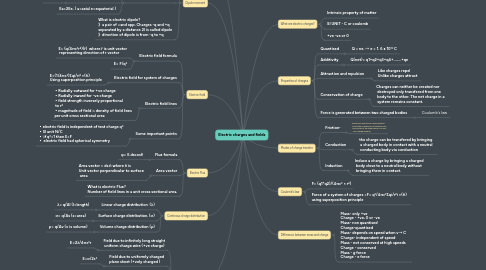# Electric charges and fieldsChaarvi M
Get Started. It's Free
or sign up with your email addressElectric charges and fields## 2. Properties of charges

### 2.1. Quantised

2.1.1. Q = ne. → e = 1. 6 x 10¹⁹ C

### 2.2. Additivity

2.2.1. Q(net)= q1+q2+q3+q4+........+qn

### 2.3. Attraction and repulsion

2.3.1. Like charges repel Unlike charges attract

### 2.4. Conservation of charge

2.4.1. Charges can neither be created nor destroyed only transfered from one body to the other. The net charge in a system remains constant.

### 2.5. Force is generated between two charged bodies

2.5.1. Coulomb's law

## 3. Modes of charge transfer

### 3.1. Friction-

3.1.1. When two objects are rubbed against each other charges are transfered from one's body to the other giving +ve and -vely charged bodies

### 3.2. Conduction

3.2.1. the charge can be transfered by bringing a charged body in contact with a neutral conducting body via conduction

### 3.3. Induction

3.3.1. Induce a charge by bringing a charged body close to a neutral body without bringing them in contact.

## 5. Gauss's laws

### 5.1. Gauss law formulae

5.1.1. Spherical shell or any random shape Φ= q/ε⁰

## 7. Electric field

### 7.1. Electric field formula

7.1.1. E= (q/4πε⁰r²)(ř) where ř is unit vector representing direction of r vector

7.1.2. E= F/q⁰

### 7.2. Electric field for system of charges

7.2.1. E=(1/4πε⁰)Σqi/ri² ×(ři) Using superposition principle

### 7.3. Electric field lines

7.3.1. ▪︎Radially outward for +ve charge ▪︎Radially inward for -ve charge ▪︎field strength inversely proportional to r² ▪︎magnitude of field ~ density of field lines per unit cross sectional area

### 7.4. Some important points

7.4.1. ▪︎electric field is independent of test charge q⁰ ▪︎SI unit N/C ▪︎ if q⁰=1 then E=F ▪︎ electric field had spherical symmetry

## 8. Dipole moment

### 8.1. Torque (τ) due to Dipole in uniform electric field

8.1.1. τ= Ρ×Εsinθ Fnet=0 in uniform electric field

### 8.2. Field of an electric dipole

8.2.1. Point on axial line

8.2.1.1. E=2kp/r³

8.2.2. Point on equatorial plane

8.2.2.1. E=kp/r³

8.2.3. Ea=2Ee. ( a=axial e=equatorial )

## 9. Electric Flux

### 9.1. Flux formula

9.1.1. φ= E.dscosθ

### 9.2. Area vector

9.2.1. Area vector = ds.ñ where ñ is Unit vector perpendicular to surface area

## 10. Continous charge distribution

### 10.1. Linear charge distribution (λ)

10.1.1. λ= q/Δl (l=length)

### 10.2. Surface charge distribution. (σ)

10.2.1. σ= q/Δs (s=area)

### 10.3. Volume charge distribution (ρ)

10.3.1. ρ= q/Δv (v is volume)

## 11. Gauss law application

### 11.1. Field due to infinitely long straight uniform charge wire (+ve charge)

11.1.1. Ε=2λ/4πε⁰r

11.2.1. E=σ/2ε⁰

### 11.3. Field due to uniformly charged thin spherical shell (Gaussian surface of radius r And sphere of radius R )

11.3.1. (case 2~r<R) E=0 as no charge is present within gaussian surface as all the charge is present on circumference of thin spherical shell

11.3.2. (case1:r>=R) E=(σ/ε⁰)(R²/r²) = q/4πε⁰r²